Скачать презентацию Acid-Base Equilibrium Chem 108 Acids taste sour

db69f7448fe9c473d479ed5f2891c940.ppt

• Количество слайдов: 54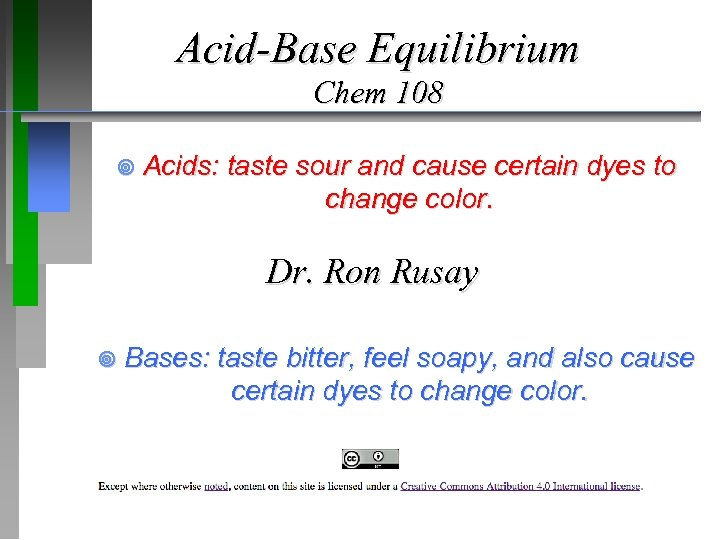Acid-Base Equilibrium Chem 108 ¥ Acids: taste sour and cause certain dyes to change color. Dr. Ron Rusay ¥ Bases: taste bitter, feel soapy, and also cause certain dyes to change color.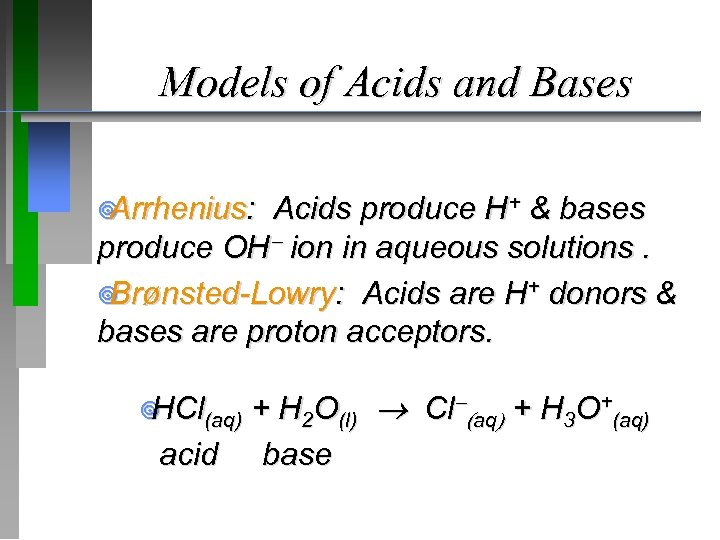Models of Acids and Bases ¥ Arrhenius: Acids produce H+ & bases produce OH ion in aqueous solutions. ¥ Brønsted-Lowry: Acids are H+ donors & bases are proton acceptors. + H 2 O(l) Cl (aq) + H 3 O+(aq) acid base ¥ HCl(aq)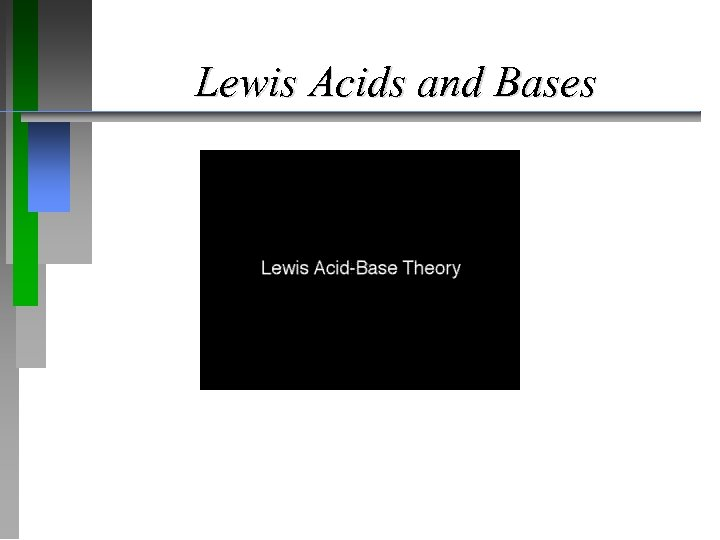Lewis Acids and Bases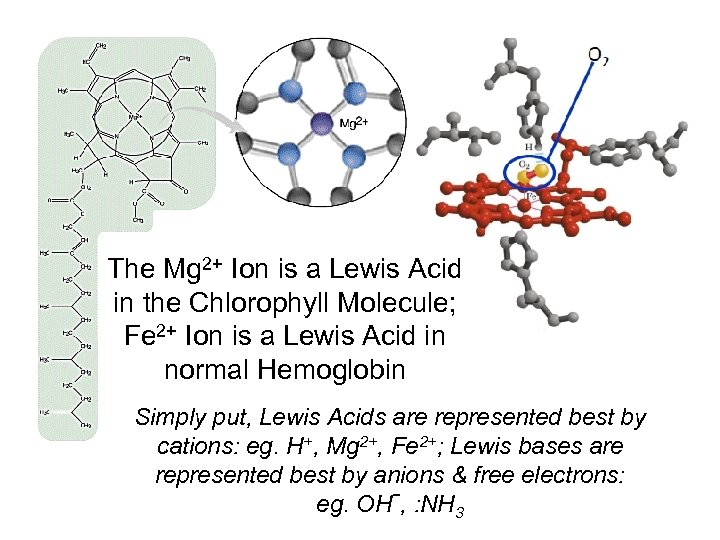The Mg 2+ Ion is a Lewis Acid in the Chlorophyll Molecule; Fe 2+ Ion is a Lewis Acid in normal Hemoglobin Simply put, Lewis Acids are represented best by cations: eg. H+, Mg 2+, Fe 2+; Lewis bases are represented best by anions & free electrons: eg. OH-, : NH 3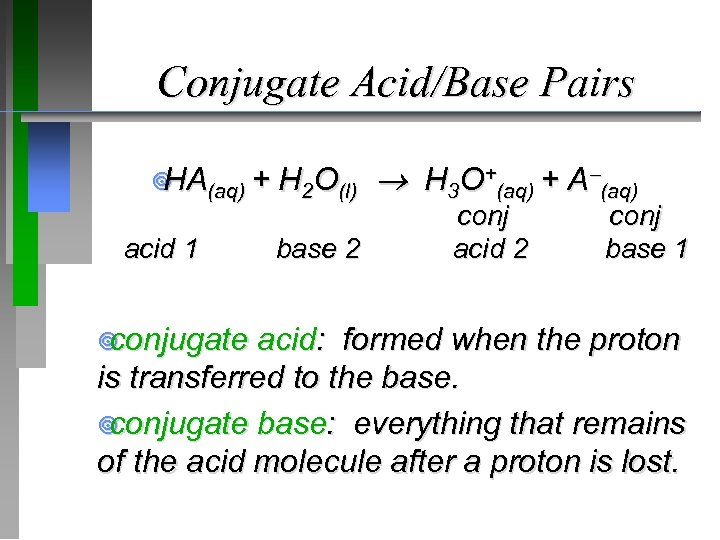Conjugate Acid/Base Pairs ¥ (aq) + HA acid 1 ¥ conjugate H 2 O(l) H 3 O+(aq) + A (aq) base 2 conj acid 2 conj base 1 acid: formed when the proton is transferred to the base. ¥ conjugate base: everything that remains of the acid molecule after a proton is lost.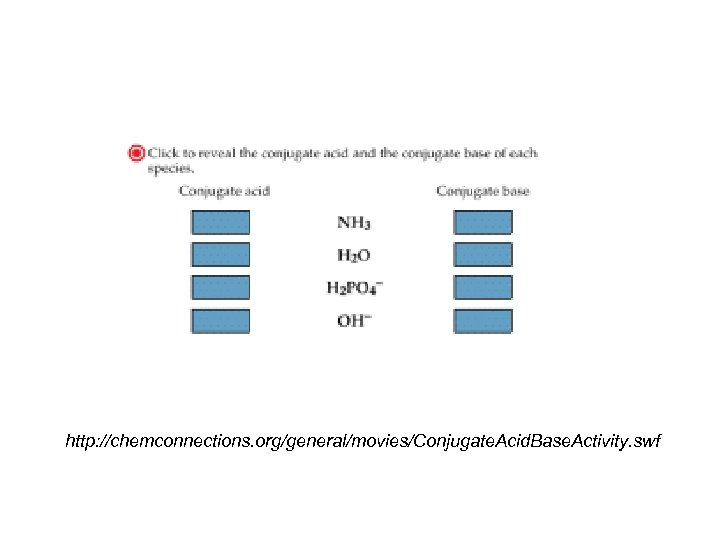http: //chemconnections. org/general/movies/Conjugate. Acid. Base. Activity. swf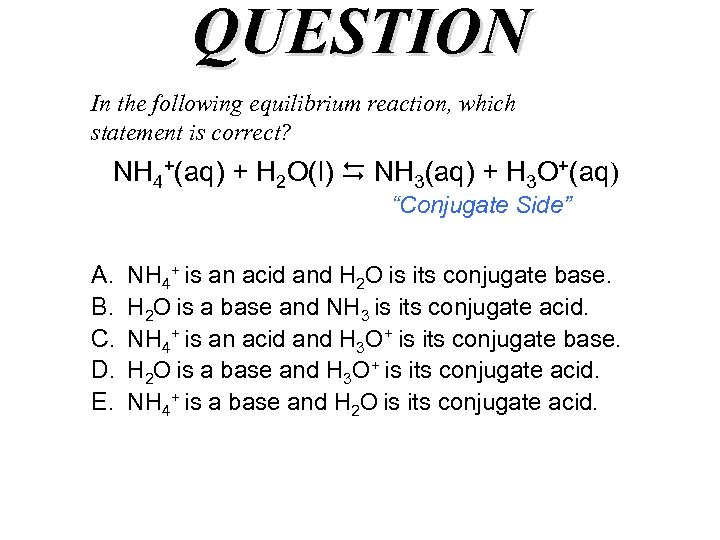QUESTION In the following equilibrium reaction, which statement is correct? NH 4+(aq) + H 2 O(l) NH 3(aq) + H 3 O+(aq) “Conjugate Side” A. B. C. D. E. NH 4+ is an acid and H 2 O is its conjugate base. H 2 O is a base and NH 3 is its conjugate acid. NH 4+ is an acid and H 3 O+ is its conjugate base. H 2 O is a base and H 3 O+ is its conjugate acid. NH 4+ is a base and H 2 O is its conjugate acid.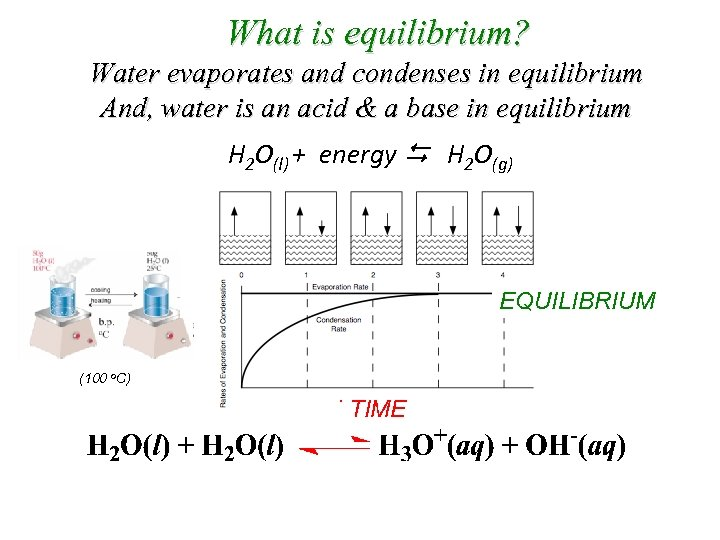What is equilibrium? Water evaporates and condenses in equilibrium And, water is an acid & a base in equilibrium H 2 O(l) + energy H 2 O(g) EQUILIBRIUM (100 o. C) TIME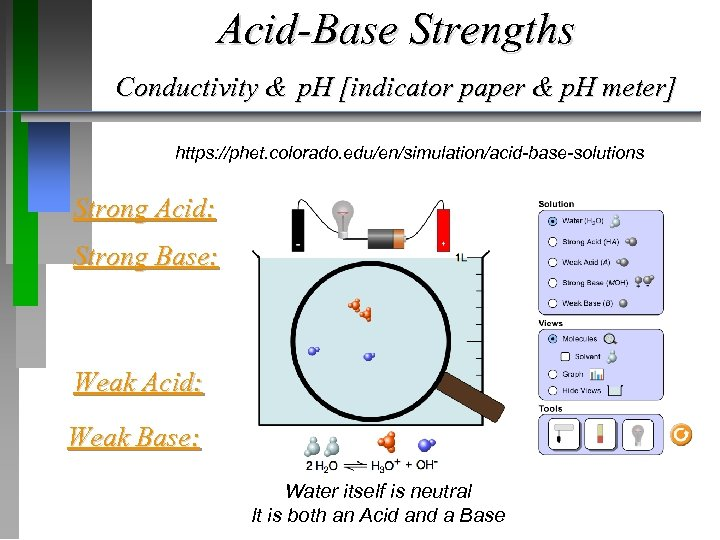Acid-Base Strengths Conductivity & p. H [indicator paper & p. H meter] https: //phet. colorado. edu/en/simulation/acid-base-solutions Strong Acid: Strong Base: Weak Acid: Weak Base: Water itself is neutral It is both an Acid and a Base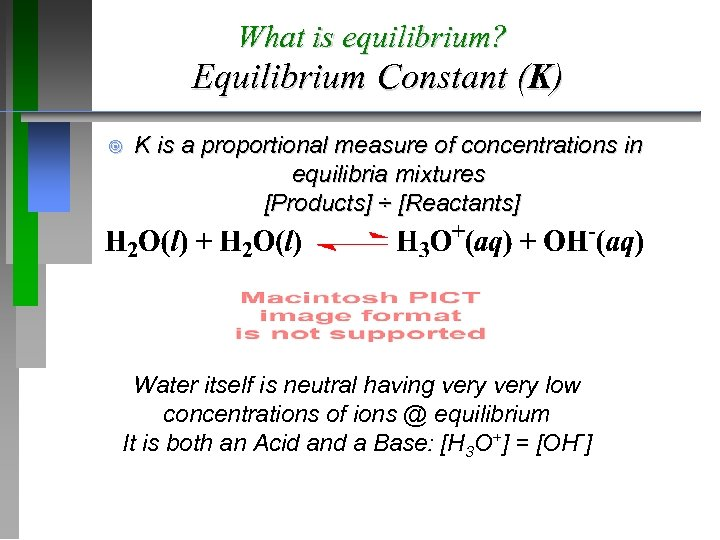What is equilibrium? Equilibrium Constant (K) ¥ K is a proportional measure of concentrations in equilibria mixtures [Products] ÷ [Reactants] Water itself is neutral having very low concentrations of ions @ equilibrium It is both an Acid and a Base: [H 3 O+] = [OH-]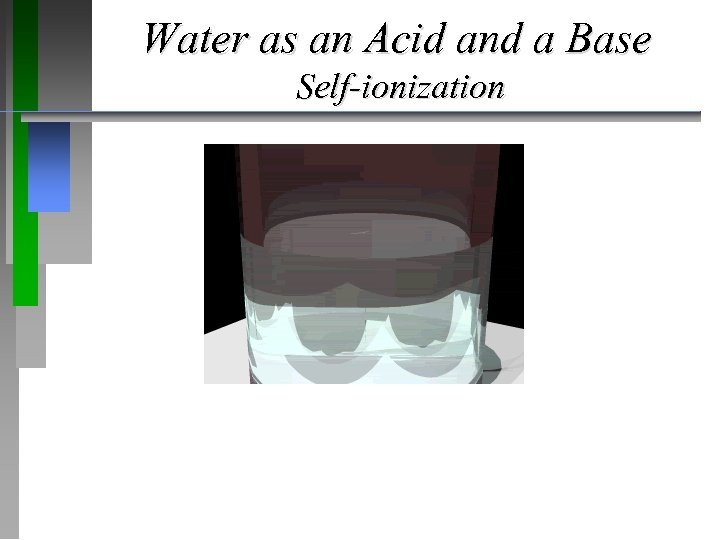Water as an Acid and a Base Self-ionization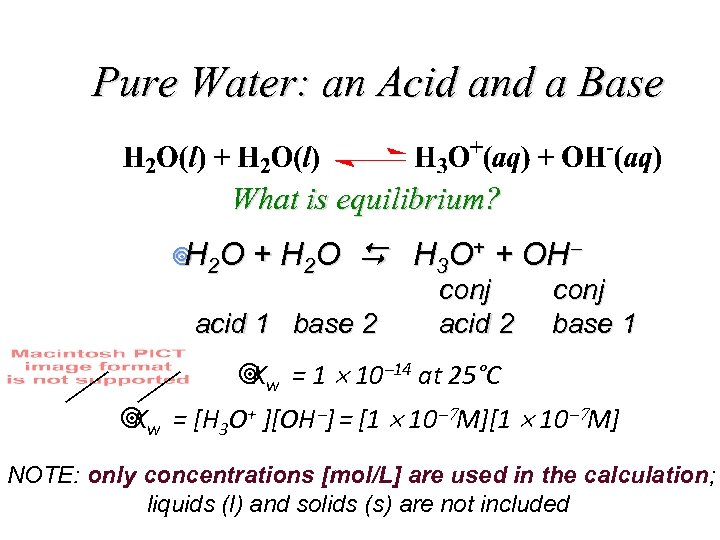Pure Water: an Acid and a Base What is equilibrium? ¥ 2 O H + H 2 O H 3 O+ + OH acid 1 base 2 conj acid 2 conj base 1 ¥ w = 1 10 14 at 25°C K ¥ w = [H 3 O+ ][OH ] = [1 10 7 M] K NOTE: only concentrations [mol/L] are used in the calculation; liquids (l) and solids (s) are not included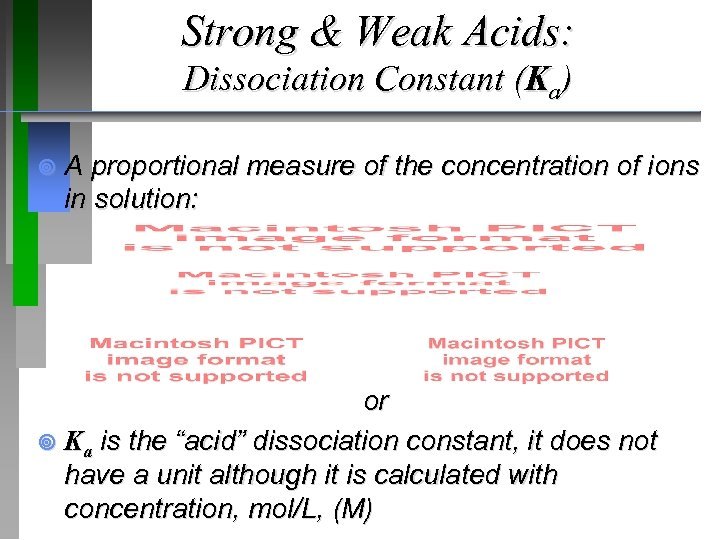Strong & Weak Acids: Dissociation Constant (Ka) ¥ A proportional measure of the concentration of ions in solution: or ¥ Ka is the “acid” dissociation constant, it does not have a unit although it is calculated with concentration, mol/L, (M)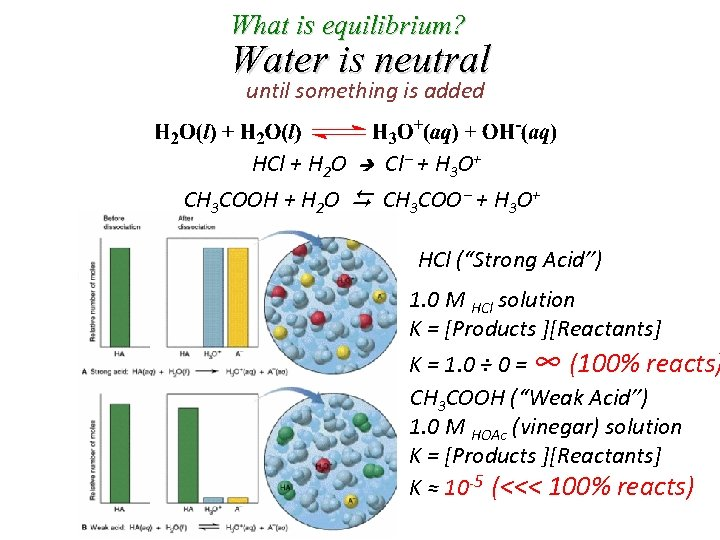What is equilibrium? Water is neutral until something is added HCl + H 2 O Cl + H 3 O+ CH 3 COOH + H 2 O CH 3 COO + H 3 O+ HCl (“Strong Acid”) 1. 0 M HCl solution K = [Products ][Reactants] K = 1. 0 ÷ 0 = ∞ (100% reacts) CH 3 COOH (“Weak Acid”) 1. 0 M HOAc (vinegar) solution K = [Products ][Reactants] K ≈ 10 -5 (<<< 100% reacts)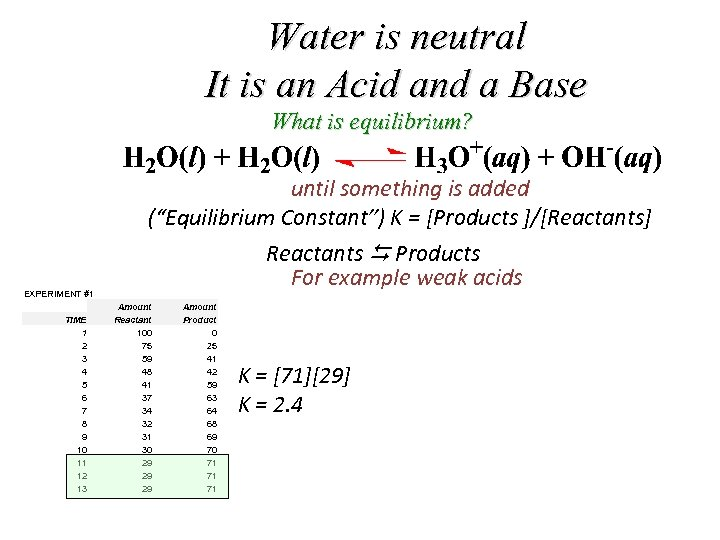Water is neutral It is an Acid and a Base What is equilibrium? until something is added (“Equilibrium Constant”) K = [Products ]/[Reactants] Reactants Products For example weak acids EXPERIMENT #1 TIME 1 2 3 4 5 6 7 8 9 10 11 12 13 Amount Reactant 100 75 59 48 41 37 34 32 31 30 29 29 29 Amount Product 0 25 41 42 59 63 64 68 69 70 71 71 71 K =  K = 2. 4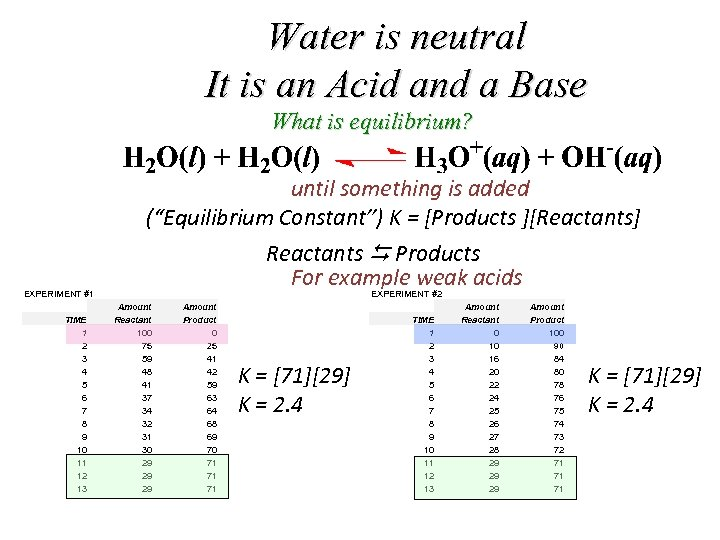Water is neutral It is an Acid and a Base What is equilibrium? until something is added (“Equilibrium Constant”) K = [Products ][Reactants] Reactants Products For example weak acids EXPERIMENT #1 TIME 1 2 3 4 5 6 7 8 9 10 11 12 13 EXPERIMENT #2 Amount Reactant 100 75 59 48 41 37 34 32 31 30 29 29 29 Amount Product 0 25 41 42 59 63 64 68 69 70 71 71 71 K =  K = 2. 4 TIME 1 2 3 4 5 6 7 8 9 10 11 12 13 Amount Reactant 0 10 16 20 22 24 25 26 27 28 29 29 29 Amount Product 100 90 84 80 78 76 75 74 73 72 71 71 71 K =  K = 2. 4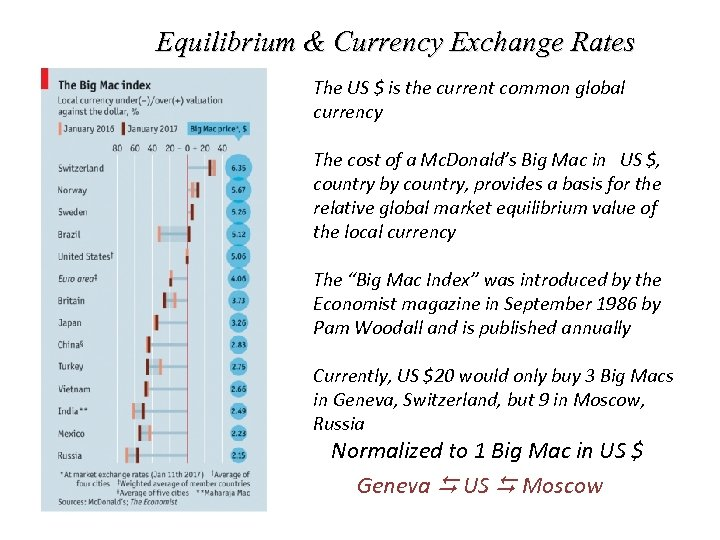Equilibrium & Currency Exchange Rates The US \$ is the current common global currency The cost of a Mc. Donald’s Big Mac in US \$, country by country, provides a basis for the relative global market equilibrium value of the local currency The “Big Mac Index” was introduced by the Economist magazine in September 1986 by Pam Woodall and is published annually Currently, US \$20 would only buy 3 Big Macs in Geneva, Switzerland, but 9 in Moscow, Russia Normalized to 1 Big Mac in US \$ Geneva US Moscow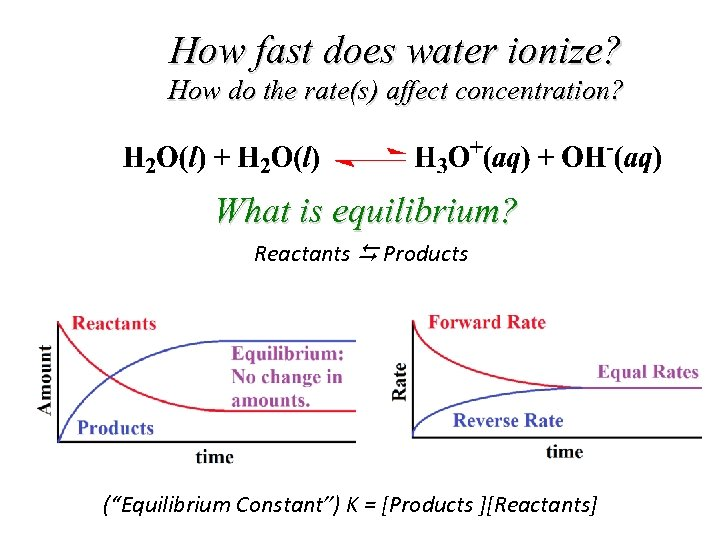How fast does water ionize? How do the rate(s) affect concentration? What is equilibrium? Reactants Products (“Equilibrium Constant”) K = [Products ][Reactants]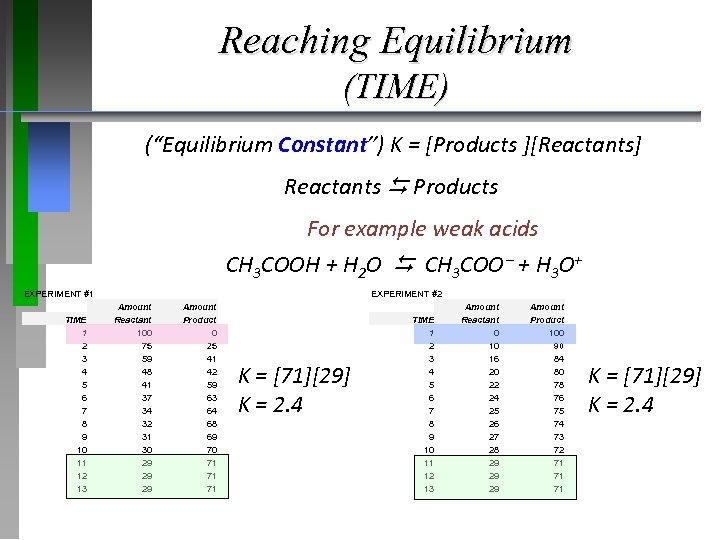Reaching Equilibrium (TIME) (“Equilibrium Constant”) K = [Products ][Reactants] Reactants Products For example weak acids CH 3 COOH + H 2 O CH 3 COO + H 3 O+ EXPERIMENT #1 TIME 1 2 3 4 5 6 7 8 9 10 11 12 13 EXPERIMENT #2 Amount Reactant 100 75 59 48 41 37 34 32 31 30 29 29 29 Amount Product 0 25 41 42 59 63 64 68 69 70 71 71 71 K =  K = 2. 4 TIME 1 2 3 4 5 6 7 8 9 10 11 12 13 Amount Reactant 0 10 16 20 22 24 25 26 27 28 29 29 29 Amount Product 100 90 84 80 78 76 75 74 73 72 71 71 71 K =  K = 2. 4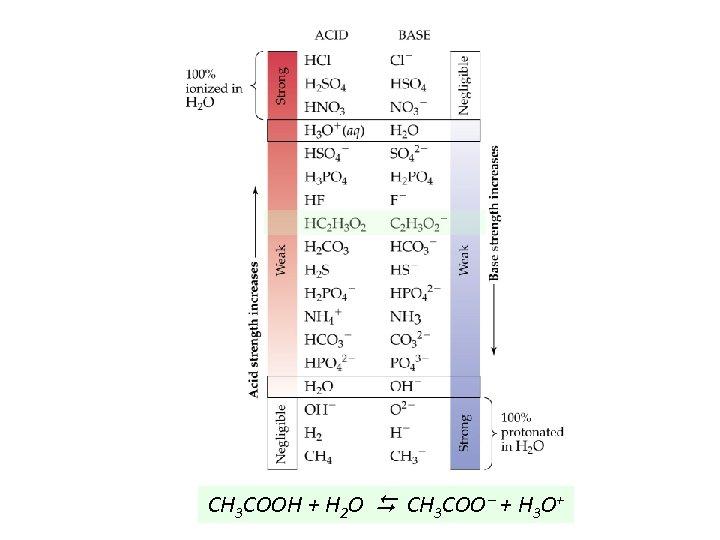CH 3 COOH + H 2 O CH 3 COO + H 3 O+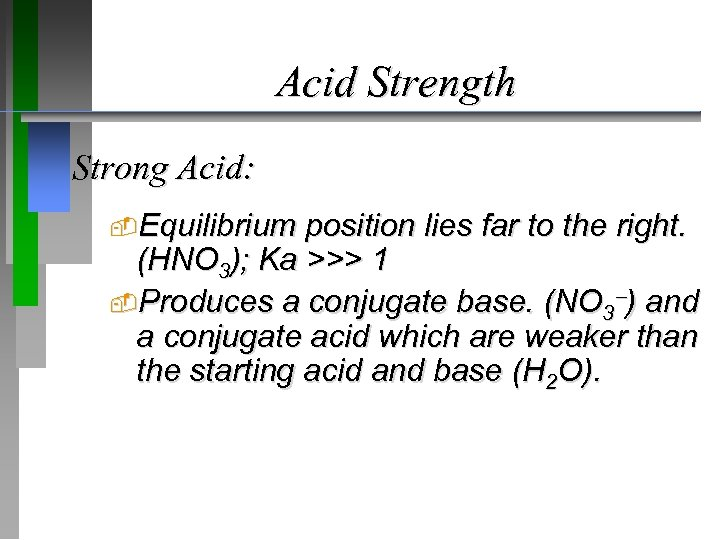Acid Strength Strong Acid: Equilibrium position lies far to the right. (HNO 3); Ka >>> 1 Produces a conjugate base. (NO 3 ) and a conjugate acid which are weaker than the starting acid and base (H 2 O).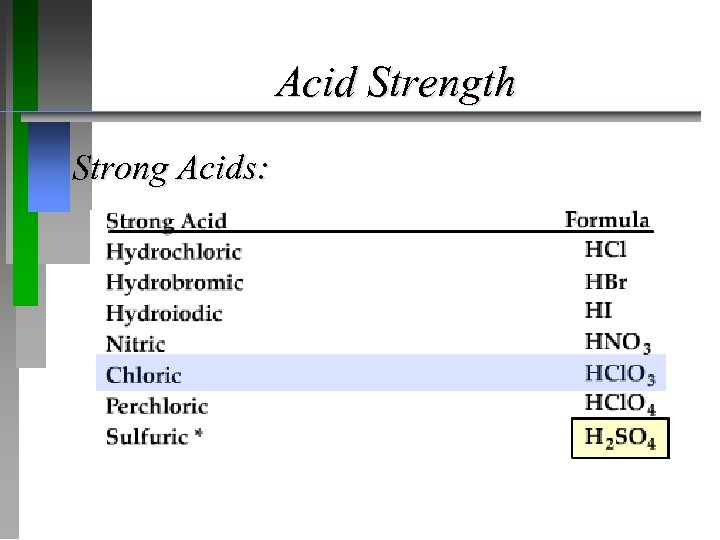Acid Strength Strong Acids: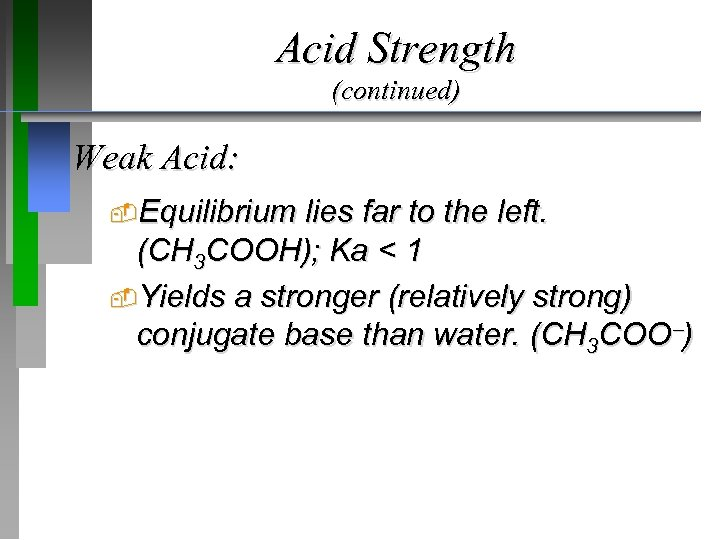Acid Strength (continued) Weak Acid: Equilibrium lies far to the left. (CH 3 COOH); Ka < 1 Yields a stronger (relatively strong) conjugate base than water. (CH 3 COO )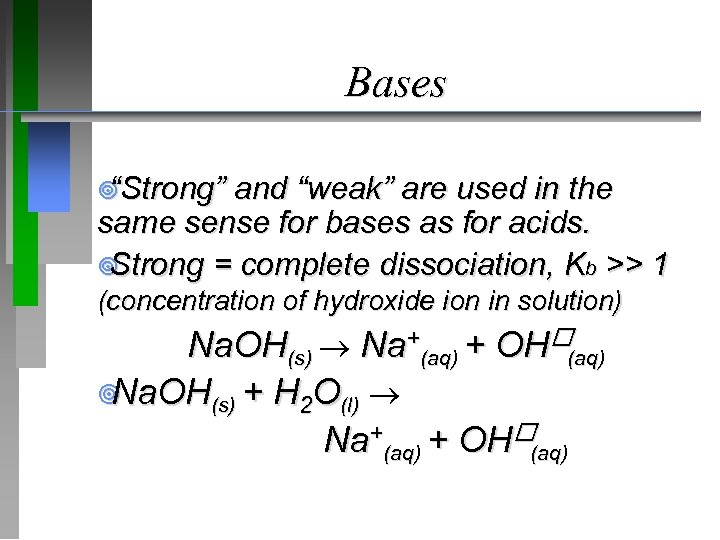Bases ¥ Strong” “ and “weak” are used in the same sense for bases as for acids. ¥ Strong = complete dissociation, Kb >> 1 (concentration of hydroxide ion in solution) Na. OH(s) Na+(aq) + OH (aq) ¥ Na. OH(s) + H 2 O(l) Na+(aq) + OH (aq)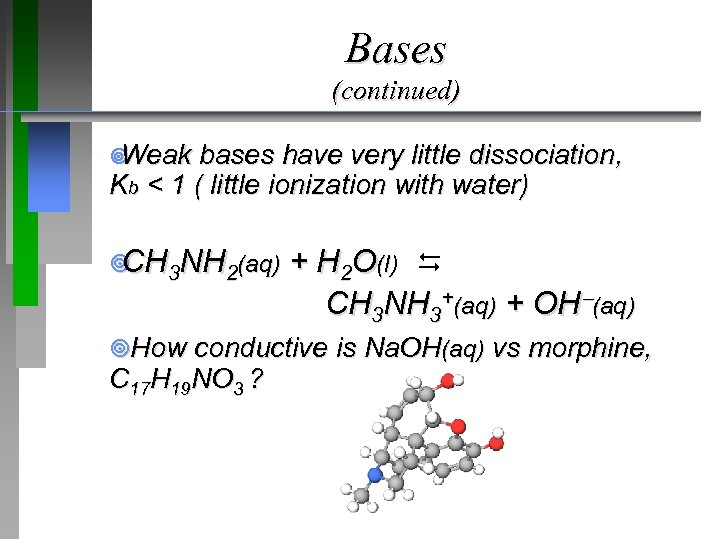Bases (continued) ¥ Weak bases have very little dissociation, Kb < 1 ( little ionization with water) ¥ 3 NH 2(aq) CH + H 2 O(l) CH 3 NH 3+(aq) + OH (aq) ¥How conductive is Na. OH(aq) vs morphine, C 17 H 19 NO 3 ?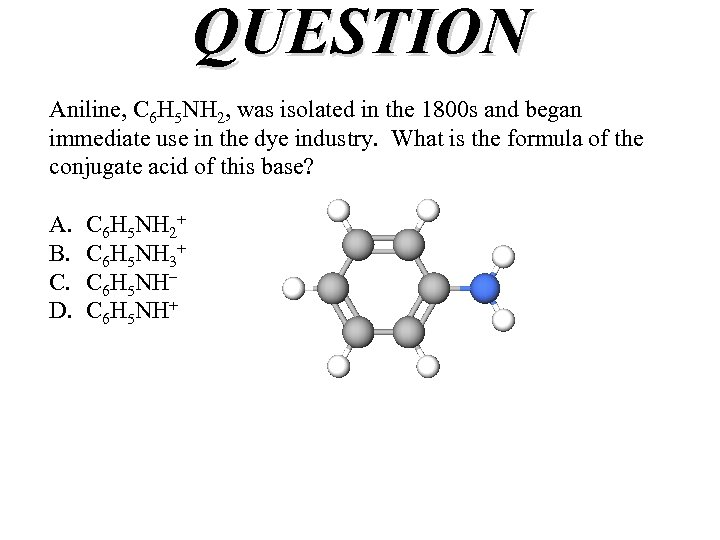QUESTION Aniline, C 6 H 5 NH 2, was isolated in the 1800 s and began immediate use in the dye industry. What is the formula of the conjugate acid of this base? A. B. C. D. C 6 H 5 NH 2+ C 6 H 5 NH 3+ C 6 H 5 NH– C 6 H 5 NH+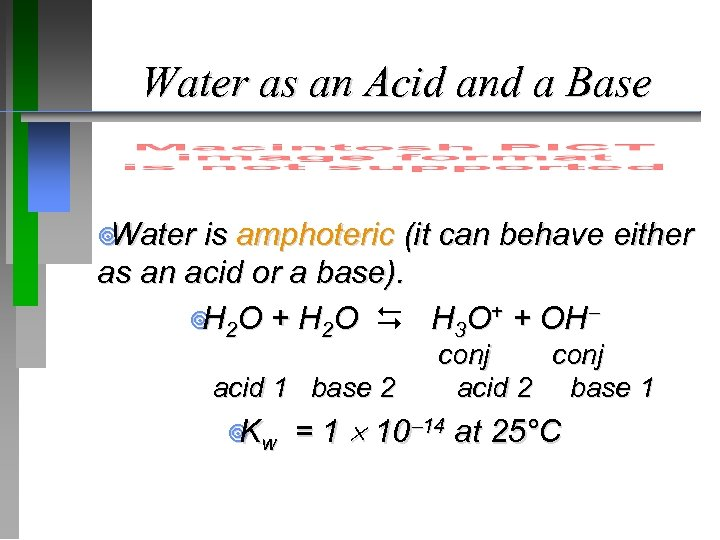Water as an Acid and a Base ¥ Water is amphoteric (it can behave either as an acid or a base). ¥ 2 O + H 2 O H 3 O+ + OH H acid 1 base 2 ¥ w K conj acid 2 base 1 = 1 10 14 at 25°C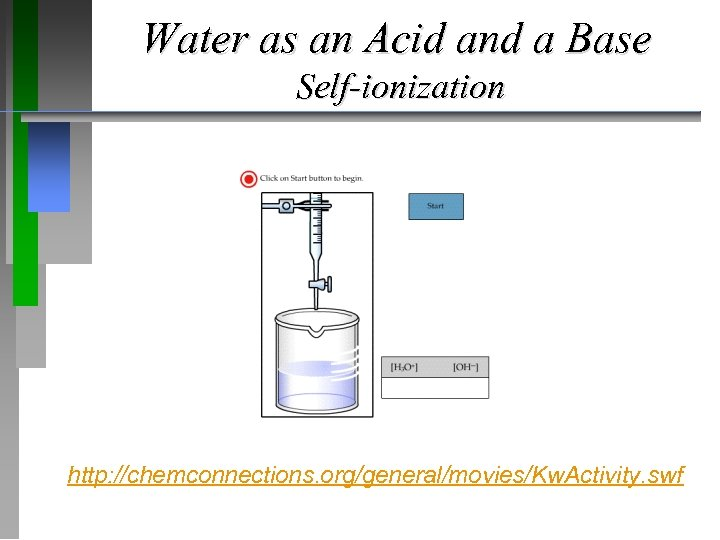Water as an Acid and a Base Self-ionization http: //chemconnections. org/general/movies/Kw. Activity. swf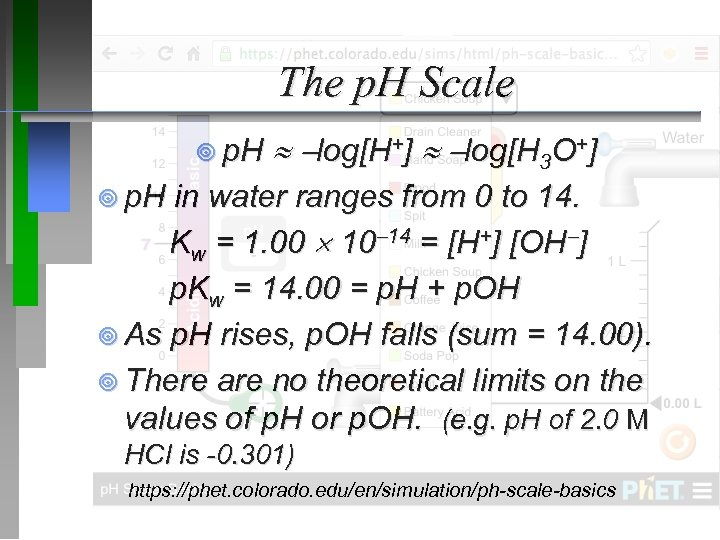The p. H Scale ¥ p. H log[H+] log[H 3 O+] ¥ p. H in water ranges from 0 to 14. Kw = 1. 00 10 14 = [H+] [OH ] p. Kw = 14. 00 = p. H + p. OH ¥ As p. H rises, p. OH falls (sum = 14. 00). ¥ There are no theoretical limits on the values of p. H or p. OH. (e. g. p. H of 2. 0 M HCl is -0. 301) https: //phet. colorado. edu/en/simulation/ph-scale-basics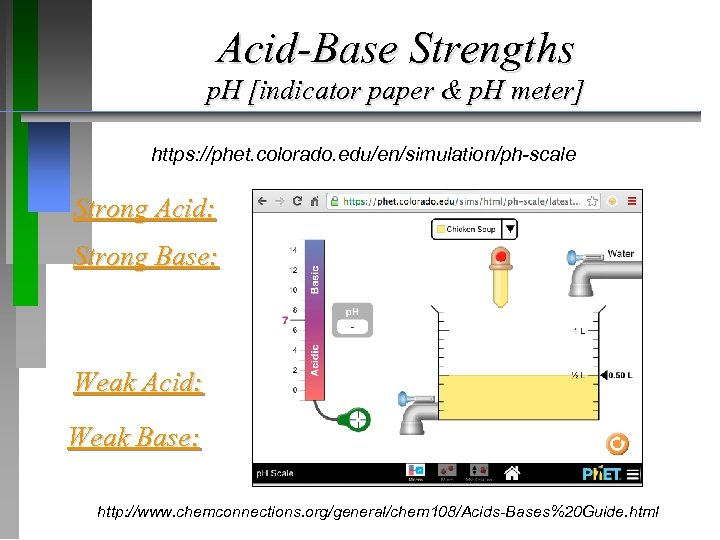Acid-Base Strengths p. H [indicator paper & p. H meter] https: //phet. colorado. edu/en/simulation/ph-scale Strong Acid: Strong Base: Weak Acid: Weak Base: http: //www. chemconnections. org/general/chem 108/Acids-Bases%20 Guide. html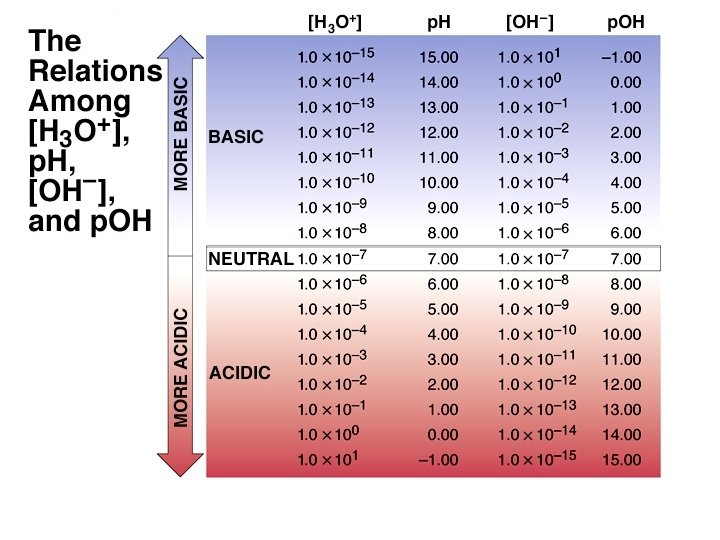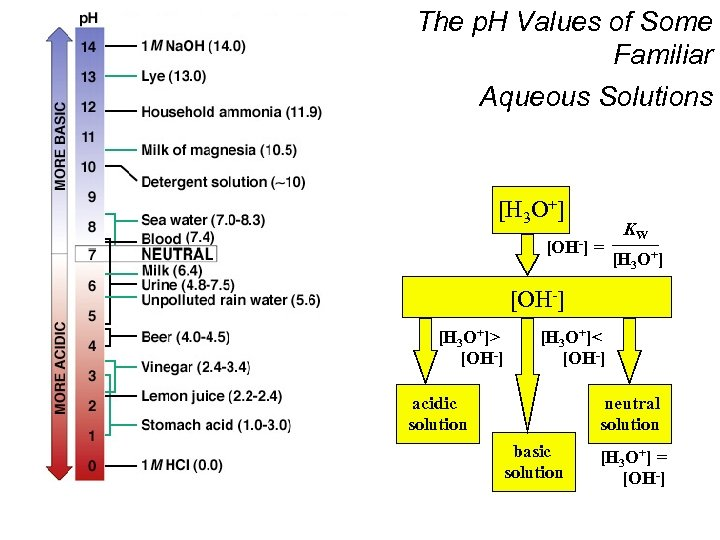The p. H Values of Some Familiar Aqueous Solutions [H 3 O+] [OH-] = KW [H 3 O+] [OH-] [H 3 O+]> [OH-] [H 3 O+]< [OH-] acidic solution neutral solution basic solution [H 3 O+] = [OH-]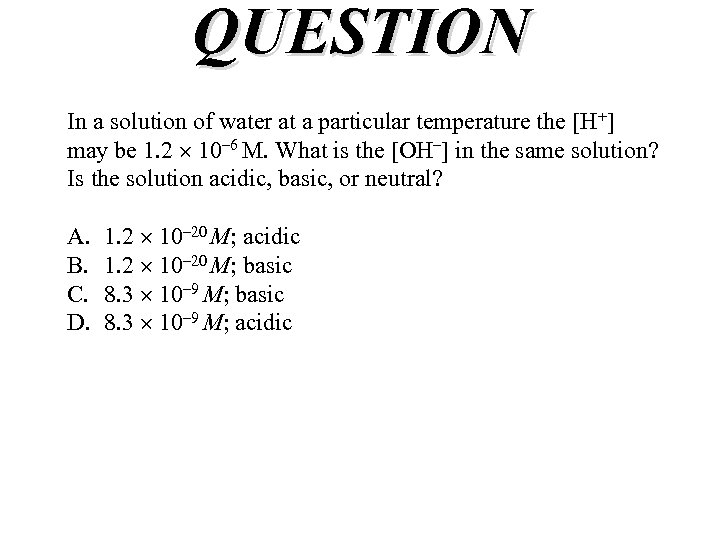QUESTION In a solution of water at a particular temperature the [H+] may be 1. 2 10– 6 M. What is the [OH–] in the same solution? Is the solution acidic, basic, or neutral? A. B. C. D. 1. 2 10– 20 M; acidic 1. 2 10– 20 M; basic 8. 3 10– 9 M; acidic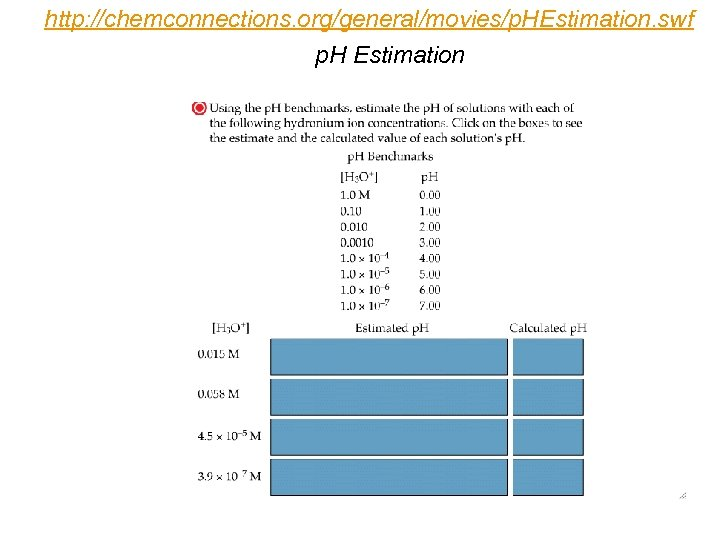http: //chemconnections. org/general/movies/p. HEstimation. swf p. H Estimation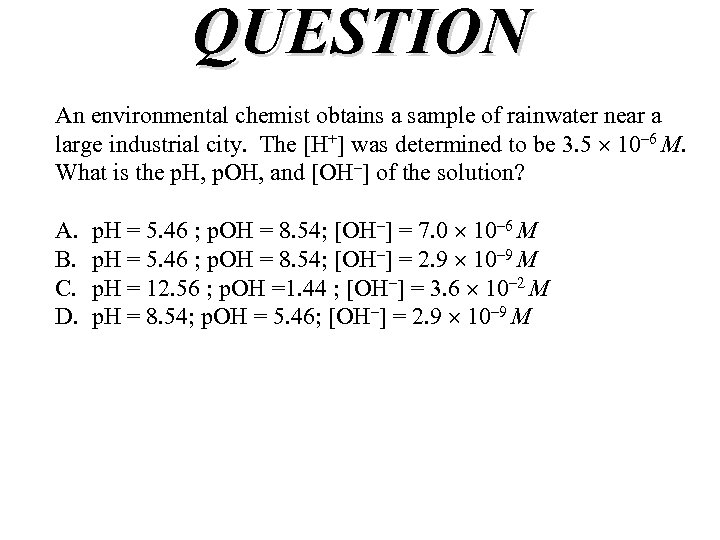QUESTION An environmental chemist obtains a sample of rainwater near a large industrial city. The [H+] was determined to be 3. 5 10– 6 M. What is the p. H, p. OH, and [OH–] of the solution? A. B. C. D. p. H = 5. 46 ; p. OH = 8. 54; [OH–] = 7. 0 10– 6 M p. H = 5. 46 ; p. OH = 8. 54; [OH–] = 2. 9 10– 9 M p. H = 12. 56 ; p. OH =1. 44 ; [OH–] = 3. 6 10– 2 M p. H = 8. 54; p. OH = 5. 46; [OH–] = 2. 9 10– 9 M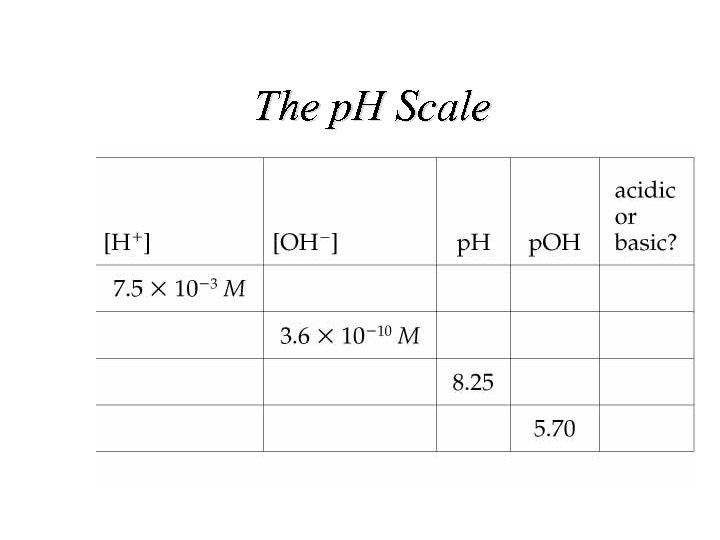The p. H Scale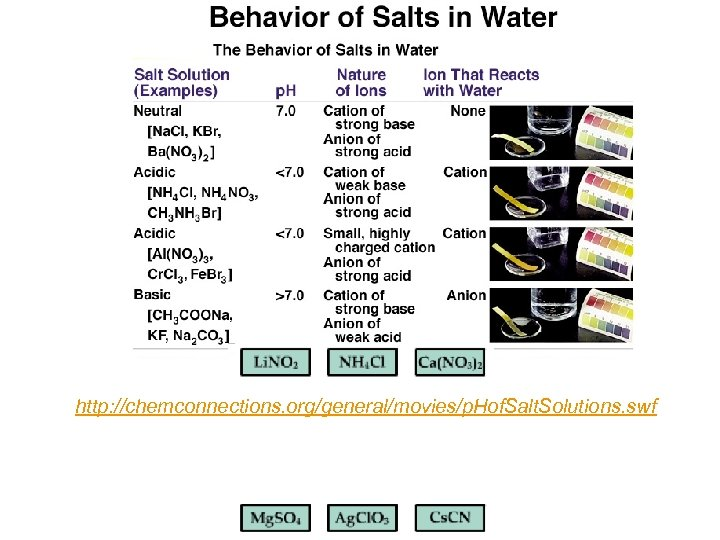http: //chemconnections. org/general/movies/p. Hof. Salt. Solutions. swf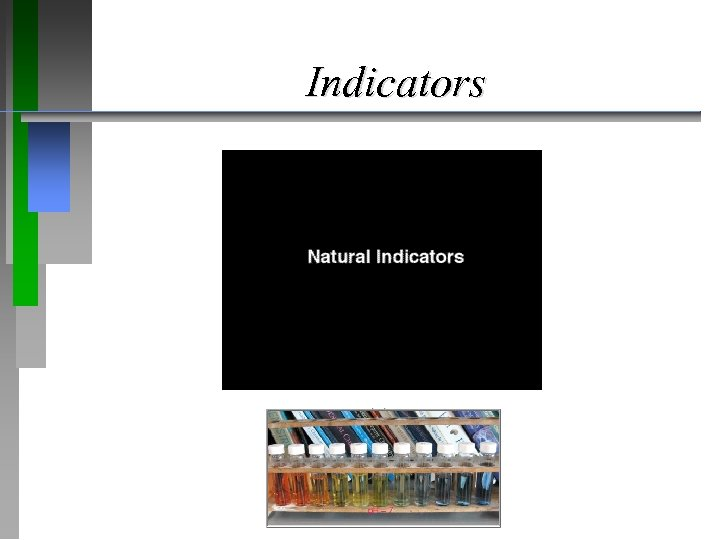Indicators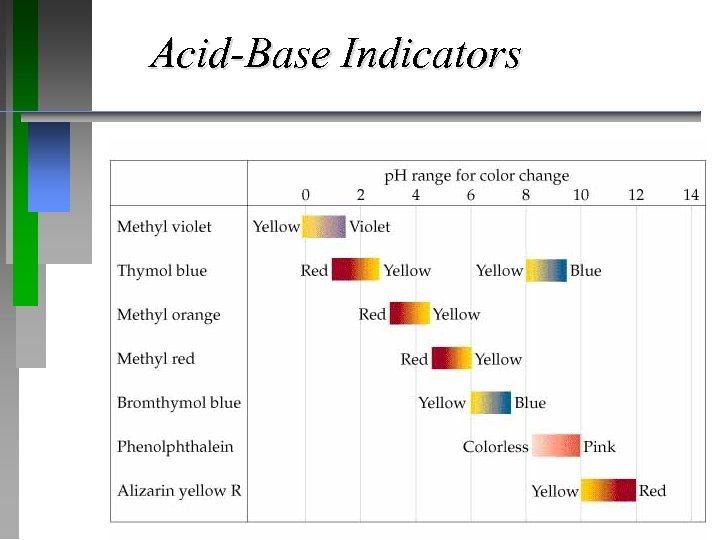Acid-Base Indicators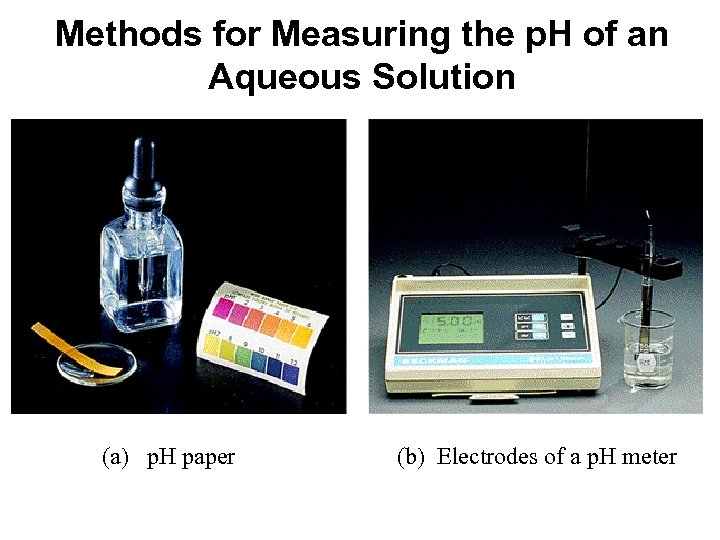Methods for Measuring the p. H of an Aqueous Solution (a) p. H paper (b) Electrodes of a p. H meter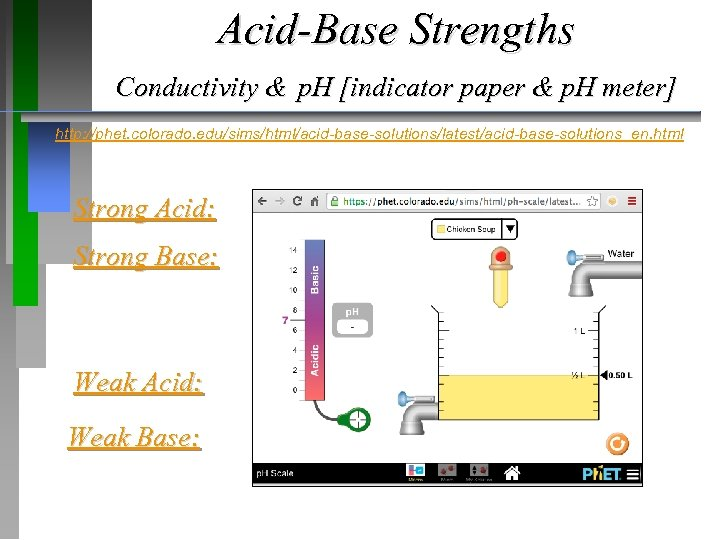Acid-Base Strengths Conductivity & p. H [indicator paper & p. H meter] http: //phet. colorado. edu/sims/html/acid-base-solutions/latest/acid-base-solutions_en. html Strong Acid: Strong Base: Weak Acid: Weak Base: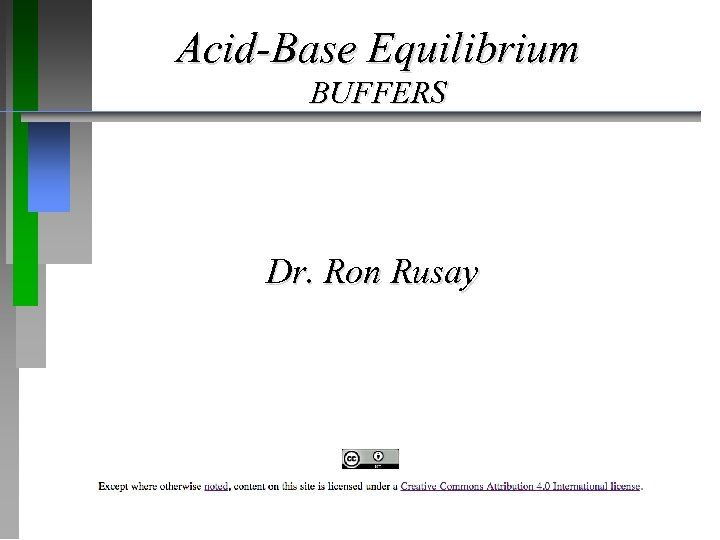Acid-Base Equilibrium BUFFERS Dr. Ron Rusay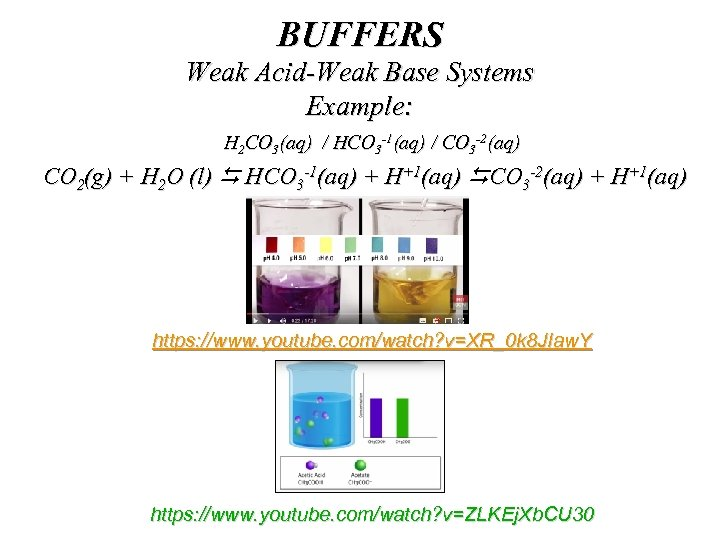BUFFERS Weak Acid-Weak Base Systems Example: H 2 CO 3(aq) / HCO 3 -1(aq) / CO 3 -2(aq) CO 2(g) + H 2 O (l) HCO 3 -1(aq) + H+1(aq) CO 3 -2(aq) + H+1(aq) https: //www. youtube. com/watch? v=XR_0 k 8 JIaw. Y https: //www. youtube. com/watch? v=ZLKEj. Xb. CU 30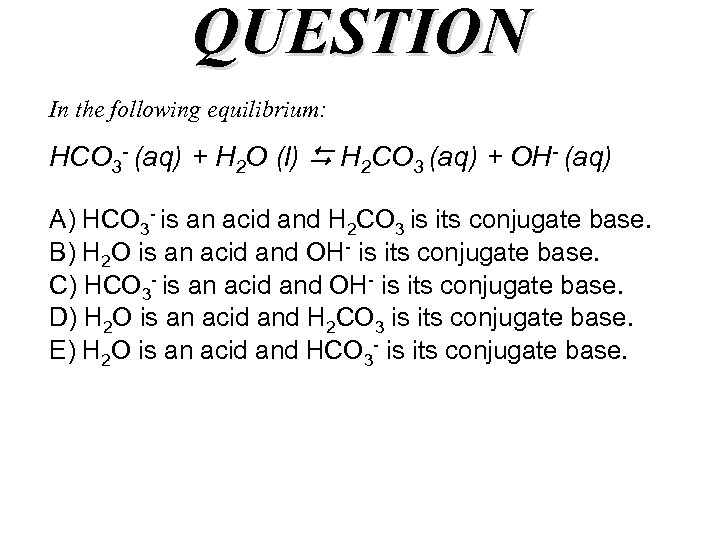QUESTION In the following equilibrium: HCO 3 - (aq) + H 2 O (l) H 2 CO 3 (aq) + OH- (aq) A) HCO 3 - is an acid and H 2 CO 3 is its conjugate base. B) H 2 O is an acid and OH- is its conjugate base. C) HCO 3 - is an acid and OH- is its conjugate base. D) H 2 O is an acid and H 2 CO 3 is its conjugate base. E) H 2 O is an acid and HCO 3 - is its conjugate base.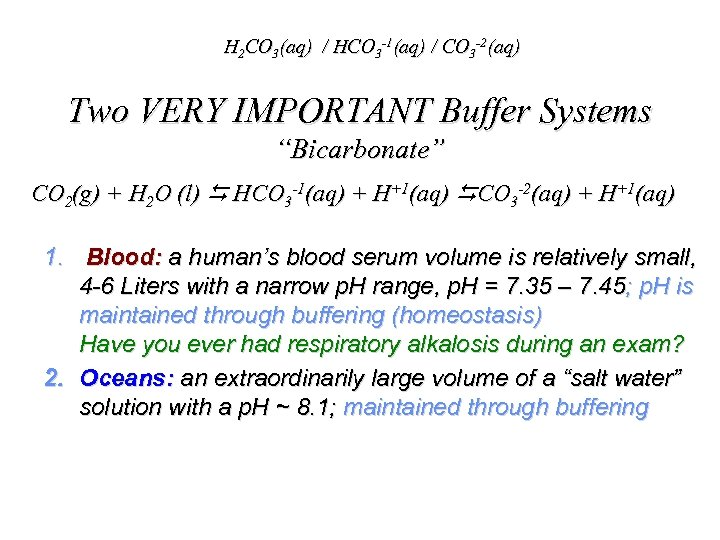H 2 CO 3(aq) / HCO 3 -1(aq) / CO 3 -2(aq) Two VERY IMPORTANT Buffer Systems “Bicarbonate” CO 2(g) + H 2 O (l) HCO 3 -1(aq) + H+1(aq) CO 3 -2(aq) + H+1(aq) 1. Blood: a human’s blood serum volume is relatively small, 4 -6 Liters with a narrow p. H range, p. H = 7. 35 – 7. 45; p. H is maintained through buffering (homeostasis) Have you ever had respiratory alkalosis during an exam? 2. Oceans: an extraordinarily large volume of a “salt water” solution with a p. H ~ 8. 1; maintained through buffering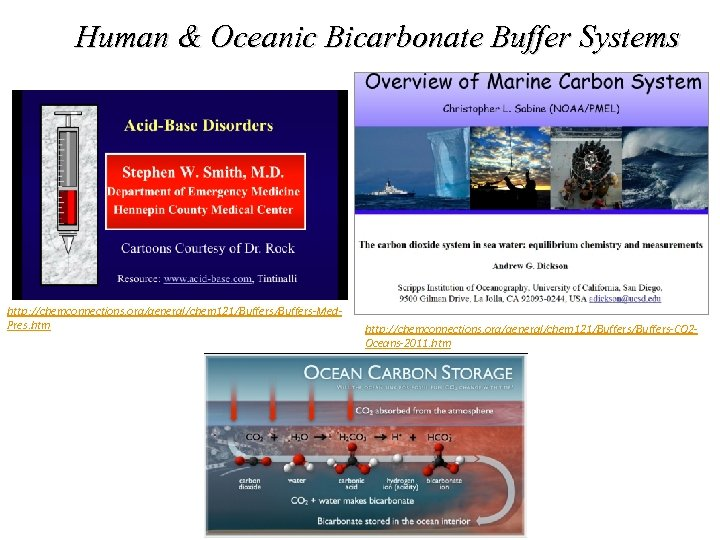Human & Oceanic Bicarbonate Buffer Systems http: //chemconnections. org/general/chem 121/Buffers-Med. Pres. htm http: //chemconnections. org/general/chem 121/Buffers-CO 2 Oceans-2011. htm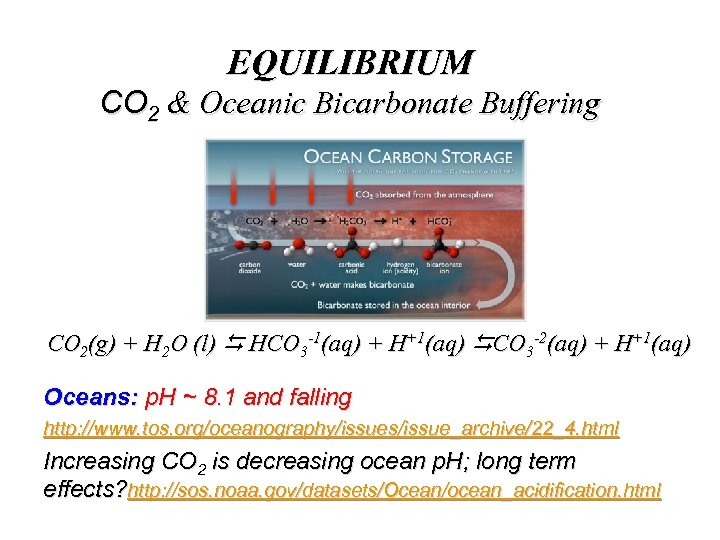EQUILIBRIUM CO 2 & Oceanic Bicarbonate Buffering CO 2(g) + H 2 O (l) HCO 3 -1(aq) + H+1(aq) CO 3 -2(aq) + H+1(aq) Oceans: p. H ~ 8. 1 and falling http: //www. tos. org/oceanography/issues/issue_archive/22_4. html Increasing CO 2 is decreasing ocean p. H; long term effects? http: //sos. noaa. gov/datasets/Ocean/ocean_acidification. html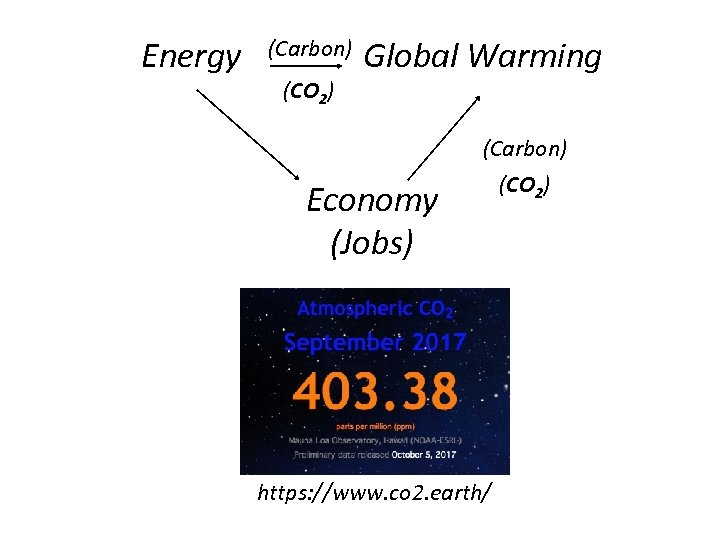Energy (Carbon) (CO 2) Global Warming (Carbon) Economy (Jobs) https: //www. co 2. earth/ (CO 2)Global Warming / Climate Change http: //chemconnections. org/IFTVET-2016/IFVET-Global%20 Warming. htmGlobal Warming, Your Carbon Footprint & Your Future http: //chemconnections. org/Global%20 Warming/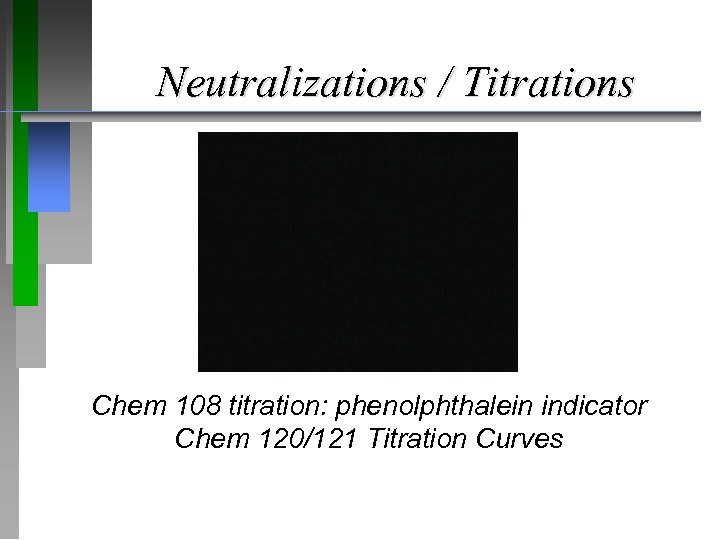Neutralizations / Titrations Chem 108 titration: phenolphthalein indicator Chem 120/121 Titration Curves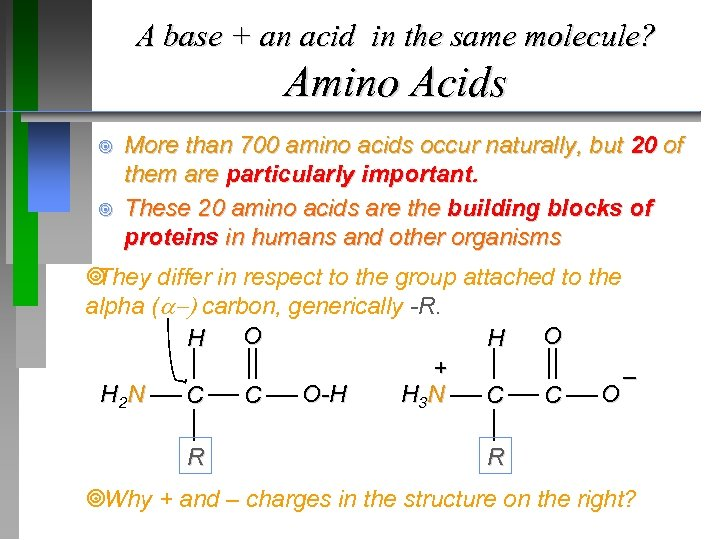A base + an acid in the same molecule? Amino Acids ¥ ¥ More than 700 amino acids occur naturally, but 20 of them are particularly important. These 20 amino acids are the building blocks of proteins in humans and other organisms ¥ They differ in respect to the group attached to the alpha (a ) carbon, generically -R. O O H H + – H 2 N O-H H 3 N O C C R R ¥Why + and – charges in the structure on the right?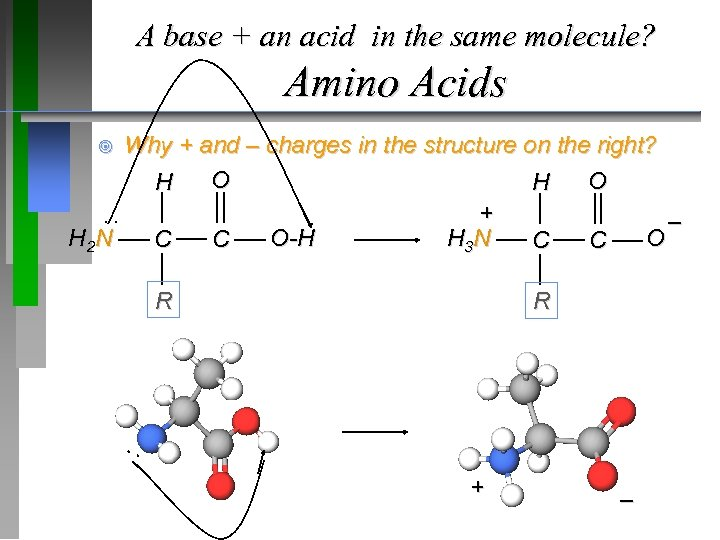A base + an acid in the same molecule? Amino Acids Why + and – charges in the structure on the right? O O H H + – H 2 N O-H H 3 N O C C ¥ : R R : + –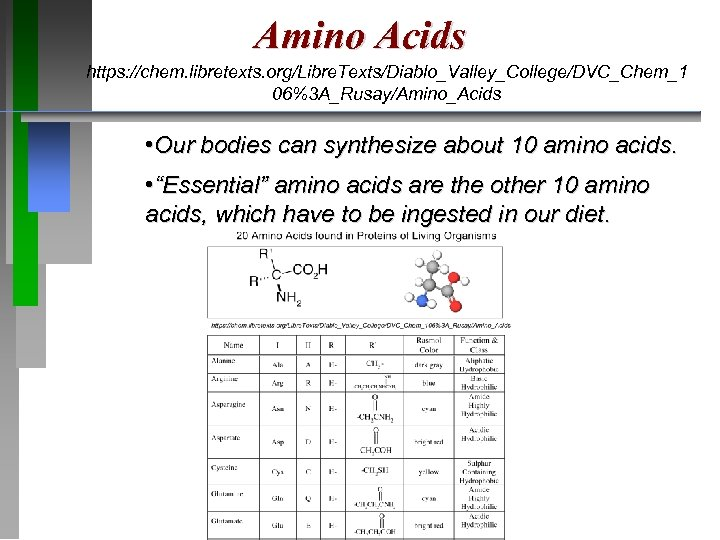Amino Acids https: //chem. libretexts. org/Libre. Texts/Diablo_Valley_College/DVC_Chem_1 06%3 A_Rusay/Amino_Acids • Our bodies can synthesize about 10 amino acids. • “Essential” amino acids are the other 10 amino acids, which have to be ingested in our diet.